Test: Fractions & Decimals

# Test: Fractions & Decimals

Test Description

## 20 Questions MCQ Test Mathematics for Class 5: NCERT | Test: Fractions & Decimals

Test: Fractions & Decimals for Railways 2023 is part of Mathematics for Class 5: NCERT preparation. The Test: Fractions & Decimals questions and answers have been prepared according to the Railways exam syllabus.The Test: Fractions & Decimals MCQs are made for Railways 2023 Exam. Find important definitions, questions, notes, meanings, examples, exercises, MCQs and online tests for Test: Fractions & Decimals below.
Solutions of Test: Fractions & Decimals questions in English are available as part of our Mathematics for Class 5: NCERT for Railways & Test: Fractions & Decimals solutions in Hindi for Mathematics for Class 5: NCERT course. Download more important topics, notes, lectures and mock test series for Railways Exam by signing up for free. Attempt Test: Fractions & Decimals | 20 questions in 20 minutes | Mock test for Railways preparation | Free important questions MCQ to study Mathematics for Class 5: NCERT for Railways Exam | Download free PDF with solutions
 1 Crore+ students have signed up on EduRev. Have you?
Test: Fractions & Decimals - Question 1

### Fraction is a _________

Test: Fractions & Decimals - Question 2

### Decimal comes from Latin word _________

Test: Fractions & Decimals - Question 3

### Mixed fraction = Nearest Natural Number + _________

Test: Fractions & Decimals - Question 4

Decimals having the same number of decimal places are called _________

Test: Fractions & Decimals - Question 5

The value of 0.25 ÷ 0.0025 × 0.025 of  2.5 is

Detailed Solution for Test: Fractions & Decimals - Question 5

we have, 0.25×0.025×0.025 of 2.5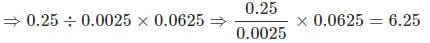Test: Fractions & Decimals - Question 6

A cricket pitch is about 364 cm. In meters it is equal to

Detailed Solution for Test: Fractions & Decimals - Question 6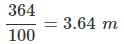Test: Fractions & Decimals - Question 7

Look at the number line and choose the right option.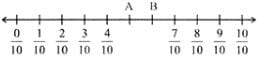A+B = ?

Detailed Solution for Test: Fractions & Decimals - Question 7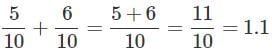Test: Fractions & Decimals - Question 8

Compare: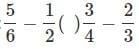Detailed Solution for Test: Fractions & Decimals - Question 8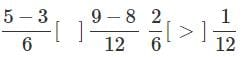Test: Fractions & Decimals - Question 9

If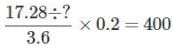then what should come in place of y ?

Detailed Solution for Test: Fractions & Decimals - Question 9

we have,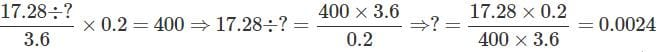Test: Fractions & Decimals - Question 10

What is the sum of shaded part?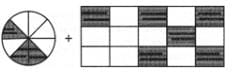Detailed Solution for Test: Fractions & Decimals - Question 10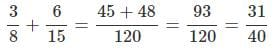Test: Fractions & Decimals - Question 11

Arrange the following decimal numbers in ascending order. 4.4, 0.44, 0.044, 0.004

Test: Fractions & Decimals - Question 12

7.5 × 7.5 + 37.5 + 2.5 × 2.5=?

Detailed Solution for Test: Fractions & Decimals - Question 12

(7.5)2 + 2 × 2.5 × 7.5 + (2.5)2 = (7.5 + 2.5)2

= (10)2 = 100

Test: Fractions & Decimals - Question 13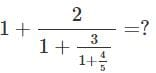Detailed Solution for Test: Fractions & Decimals - Question 13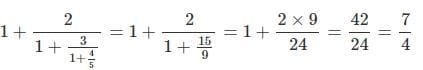Test: Fractions & Decimals - Question 14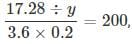then what should come in place of y?

Detailed Solution for Test: Fractions & Decimals - Question 14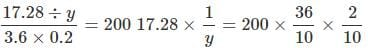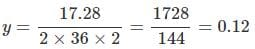Test: Fractions & Decimals - Question 15

Nitu did 1/2 of the work yesterday and one-third of the work today. How much work will she have to do tomorrow to complete the work?

Detailed Solution for Test: Fractions & Decimals - Question 15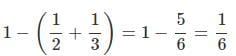Test: Fractions & Decimals - Question 16

25 litres and 25 ml is equal to

Detailed Solution for Test: Fractions & Decimals - Question 16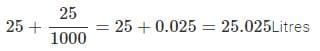Test: Fractions & Decimals - Question 17

The heights of Vicky, Bittu, Rohit and Abhishek are 1.61m, 162 cm, 1600 mm and 1640 mm respectively. Who is the tallest of them all?

Detailed Solution for Test: Fractions & Decimals - Question 17

Vicky → 1.61m Bittu →1.62m Rohit → 1.6m Abhishek →  1.64m

Test: Fractions & Decimals - Question 18

Ayush, Satyam and Mohak bought 8.5 litres, 7.25 litres and 9.4 litres milk respectively from a milk booth. If there was 30 litres of Milk in booth, find the quantity of milk left.

Detailed Solution for Test: Fractions & Decimals - Question 18

Total milk bought =8.5l+7.25l+9.4l=25.15l

∴ Milk left in booth = 30l−25.15l = 4.850 liters

Test: Fractions & Decimals - Question 19

Ravindra Jadeja took 15 wickets for 330 runs, then his average score per wicket is

Detailed Solution for Test: Fractions & Decimals - Question 19

15 wickets= 330 runs 1 wickets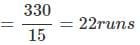Test: Fractions & Decimals - Question 20

Detailed Solution for Test: Fractions & Decimals - Question 20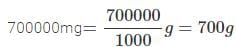## Mathematics for Class 5: NCERT

36 videos|73 docs|45 tests
 Use Code STAYHOME200 and get INR 200 additional OFF Use Coupon Code
Information about Test: Fractions & Decimals Page
In this test you can find the Exam questions for Test: Fractions & Decimals solved & explained in the simplest way possible. Besides giving Questions and answers for Test: Fractions & Decimals, EduRev gives you an ample number of Online tests for practice

## Mathematics for Class 5: NCERT

36 videos|73 docs|45 tests